Question

# 1. Explain why the Solow model with population growth and human capital helps to better understand...

1. Explain why the Solow model with population growth and human capital
helps to better understand the differences in growth, the catch-up phenomenon for
developing countries and differences in GDP between countries. Also give an example of
phenomenon that the model cannot explain.

The relationship between population growth and economic growth is controversial. This article draws on historical data to chart the links between population growth, growth in per capita output, and overall economic growth over the past 200 years. Low population growth in high-income countries is likely to create social and economic problems while high population growth in low-income countries may slow their development. International migration could help to adjust these imbalances but is opposed by many. Drawing on economic analyses of inequality, it appears that lower population growth and limited migration may contribute to increased national and global economic inequality.

If population growth and per capita GDP growth are completely independent, higher population growth rates would clearly lead to higher economic growth rates. It would still be true that, as noted by Piketty (2014), only the growth in per capita GDP would give rise to improvements in economic well-being. On the other hand, if population growth affects per capita output growth, higher population growth rates would contribute to either higher or lower overall economic growth depending on the nature of its effects on per capita GDP. For the world as a whole, over the period 1990 to 2015, the correlation between population growth and real per capita GDP growth, based on World Bank (2017) data, was −0.1849 suggesting that these two variables were uncorrelated during that period. Simple correlation, of course, tells us very little about the actual relationship between these variables. It turns out that economists have developed theoretical arguments supporting both the idea that population growth slows growth in per capita output and the opposite idea that population growth stimulates greater economic growth. Thomas Malthus (1993) developed one of the earliest and best known theories showing that population growth has a negative effect on well-being. He believed that population has a tendency to grow more rapidly than food supplies so that population reductions through various types of misery are always required to keep the number of people at a level consistent with the amount of food available. The implication of Malthus’s model is that average incomes will always be driven down by population growth to a level that is just adequate for the population’s subsistence.

A major purpose of Malthus’s essay was to argue against the English Poor Laws. He suggested that trying to increase the well-being of the poor was an exercise in futility as higher incomes would lead to population increases that would drive incomes back down to the subsistence level. This understanding represented an accurate image of the past but missed the boat entirely for the future. From 1000 to 1820, average annual population growth in England was about 0.29% while per capita GDP growth averaged 0.12% for an overall average annual economic growth rate of 0.41% according to data from World Economics (2016). With the Industrial Revolution, however, both income and population growth began to increase as did the supplies of food. Growth in global agricultural output has been faster than world population growth over the past two centuries (Peterson, 2009) and real per capita GDP in England has increased more than 11-fold since 1820 (The Maddison Project, 2013). The fact that technological innovations have allowed incomes to rise well above the subsistence levels familiar to Malthus does not mean, however, that the question of how population growth affects growth in per capita output is resolved. It is still possible that growth in output would have been greater if population growth rates had been somewhat lower. In fact, population growth in the United Kingdom between 1820 and 2010 was moderately higher at 0.57% than was the case for the previous 820 years while annual growth in per capita GDP was substantially more rapid at 1.28% after 1820 (World Economics, 2016 and The Maddison Project, 2013).

Malthusian perspectives on the effects of population growth on social and economic well-being were revived by Paul Ehrlich (1968) and others in the latter part of the 20th century when population growth rates reached very high levels, primarily in low-income countries. The concern of these writers was that world population would reach a level that would overwhelm the capacity of the earth and its resources to generate the food and other goods needed for human life. Many felt that both population growth and economic growth needed to be scaled back or eliminated entirely to avoid an existential crisis. Other authors argued that fears about population growth were overblown suggesting that population growth would stimulate technological innovations that would allow food production to keep up with the growing population (Boserup, 1965) or that larger populations would result in more brains that could be applied to solving any resource problems that might arise (Simon, 1981). In 1980, Julian Simon and Ehrlich made a famous bet on the future prices of five mineral commodities over the following 10 years with Ehrlich predicting rising prices as the world population exploded while Simon forecast the opposite as clever humans found ways to overcome resource constraints. Simon won the bet as the commodity prices fell substantially while the world added 800 million people to its population (Sabin, 2014). As population growth rates have begun to fall in recent years, the possibility that food and natural resources will be exhausted by a larger population seems to be less of a preoccupation than the more likely danger that continued use of the earth’s resources at current rates will lead to climate change and other environmental problems.

#### Earn Coins

Coins can be redeemed for fabulous gifts.

Similar Homework Help Questions
• ### The solow growth model does a pretty good job of explaining which of the following?

The solow growth model does a pretty good job of explaining which of the following? differences in deficit-GDP ratios among countries differences in savings rates between countries differences in output-labor ratios between countries differences in capital-labor ratio among countries

• ### Consider the Solow growth model with depreciation rate and population growth rate n. The equation of...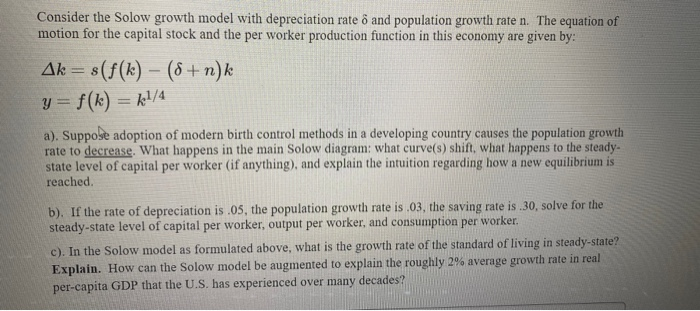Consider the Solow growth model with depreciation rate and population growth rate n. The equation of motion for the capital stock and the per worker production function in this economy are given by: Ak= s(f(k) - (8 + n) k y= f(k) = k1/4 a). Suppose adoption of modern birth control methods in a developing country causes the population growth rate to decrease. What happens in the main Solow diagram: what curve(s) shin, what happens to the steady- state level...

• ### 1. Consider the simple version of the Solow Growth Model discussed in class summarized by these...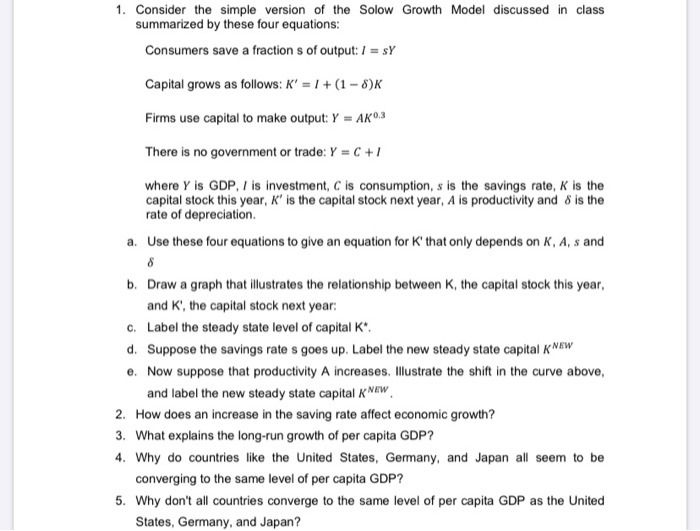1. Consider the simple version of the Solow Growth Model discussed in class summarized by these four equations: Consumers save a fraction s of output: 1 = sy Capital grows as follows: K' = 1 + (1 - 8)K Firms use capital to make output: Y = AK 0.3 There is no government or trade: Y = C+/ where Y is GDP, / is investment, C is consumption, s is the savings rate, K is the capital stock this year,...

• ### 1. Exercise 1. Predicting steady states and growth rates from Solow Model In this exercise, assume...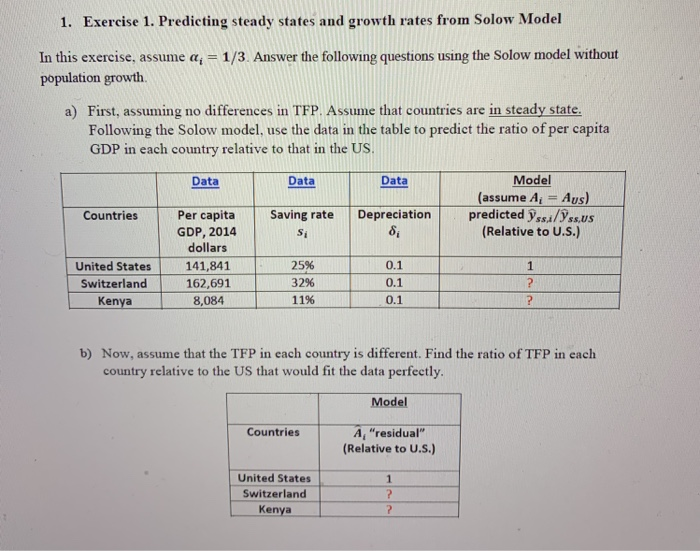1. Exercise 1. Predicting steady states and growth rates from Solow Model In this exercise, assume a, -1/3. Answer the following questions using the Solow model without population growth a) First, assuming no differences in TFP. Assume that countries are in steady state. Following the Solow model, use the data in the table to predict the ratio of per capita GDP in each country relative to that in the US. Data Data Data Countries Saving rate Model (assume A =...

• ### 1. Exercise 1. Predicting steady states and growth rates from Solow Model In this exercise, assume a = 1/3. Answer...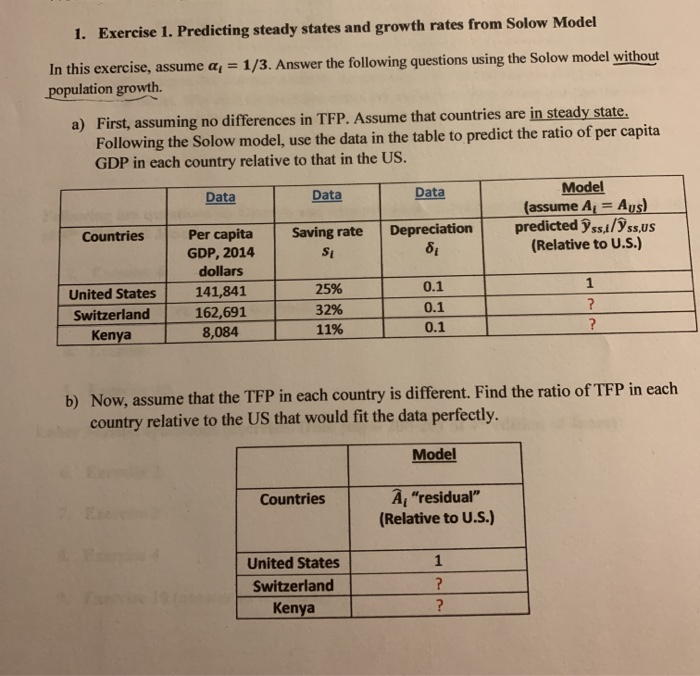1. Exercise 1. Predicting steady states and growth rates from Solow Model In this exercise, assume a = 1/3. Answer the following questions using the Solow model without population growth. a) First, assuming no differences in TFP. Assume that countries are in steady state. Following the Solow model, use the data in the table to predict the ratio of per capita GDP in each country relative to that in the US. Data Data Data Model (assume A = Aus) predicted...

• ### Describe 1. Should poor countries grow faster than rich in a Solow model? Explain 2. Explain...

Describe 1. Should poor countries grow faster than rich in a Solow model? Explain 2. Explain how changes in i. population growth rate, ii. saving rate, iii. technology growth rate change the stationary state in a Solow growth model.

• ### In the Solow model with a positive rate of population growth n and technological progress z,...

In the Solow model with a positive rate of population growth n and technological progress z, the steady state level of total real output Y grows at the rate: a. n. b. zero. c. z. d. n + z. In the Solow model with a positive rate of population growth n and technological progress z, the steady state level of per worker real output y grows at the rate: a. n. b. zero. c. z. d. n + z. In...

• ### In the Solow growth model with technological progress (and diminishing marginal returns to capital), explain the...

In the Solow growth model with technological progress (and diminishing marginal returns to capital), explain the steady-state growth rates for: a. Capital per effective worker b. Output per effective worker c. Output per worker d. Total output

• ### Consider a version of the Solow model where the population growth rate is 0.05. There is...

Consider a version of the Solow model where the population growth rate is 0.05. There is no technological progress. Capital depreciates at rate ? each period and a fraction ? of income is invested in physical capital every period. Assume that the production function is given by: ?t = ?t1/2 ?t1/2 where ?t is output, ?t is capital and ?t is labour. a. Derive an expression for the accumulation of capital per worker in this economy, i.e. ∆?t+1 where ?t...

• ### 11. In the Solow model the key driver of economic growth is a) accumulation of human...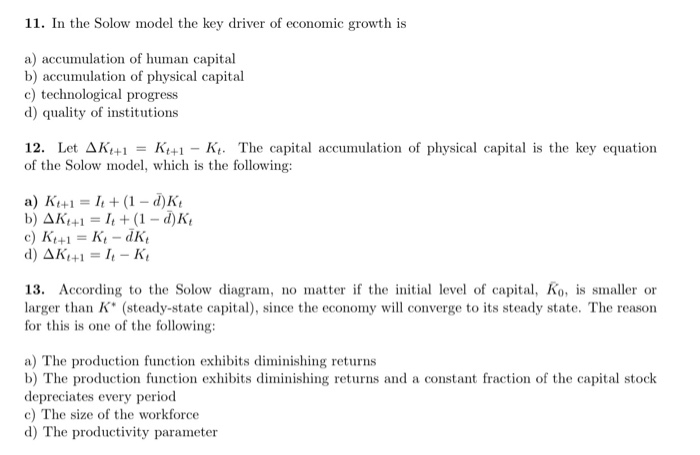11. In the Solow model the key driver of economic growth is a) accumulation of human capital b) accumulation of physical capital c) technological progress d) quality of institutions Kt+1-K The capital accumulation of physical capital is the key equation 12. Let AK+1 of the Solow model, which is the following a) Kt+1 +(1- d)K b) AK41= I+(1 - d)K c) Kt41 Ki-dK d)AK1I- K 13. According to the Solow diagram, no matter if the initial level of capital, Ko,...